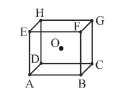# NEET Chemistry The Solid State Questions Solved

A body centred cubic arrangement is shown : O is the body centre; A, B, C, D,......., H are the corners. What is the magnitude of the angle AOB ?(A) $120°$

(B) $109°28\text{'}$

(C) $104°31\text{'}$

(D) $70°32\text{'}$

Concept Videos :-

#9 | FCC Unit Cell: Packing Fraction
#20 | Rank of HP Unit Cell
#21 | Packing Fraction of HP Unit Cell
#24 | Density of Unit Cell

Concept Questions :-

Density/Formula/packing fraction/ Semiconductors